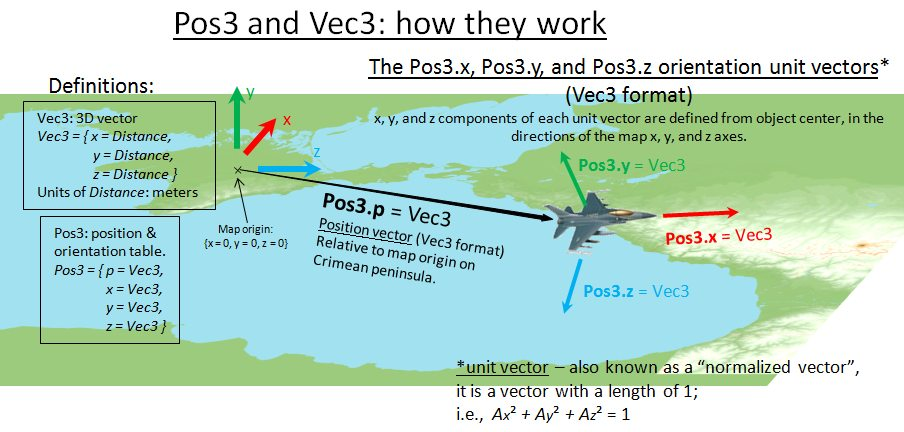Basic terms and types

Home > Support > Technical questions > DCS: World Scripting Engine > Part 1 > Basic terms and types

Basic terms and types

Basic terms and types

Enum

Enumeration types are given as

EnumType = enum EnumTable

This means the EnumType is enum type. Constants are stored in EnumTable table. It doesn't matter what is the real type of the EnumType. Mission developer must NEVER operate constant values! Only constant names must be used!

Array

Given as

ArrayType = array of ElementType

This means ArrayType is table that contains ElementType

ArrayType = {
 = ElementType,
 = ElementType,
...
[N] = ElementType
}

Map

Given as

MapType = map KeyType, ElementType

This means MapType is table that contains ElementType each with key of type KeyType.

MapType = {
[KeyType] = ElementType,
[KeyType] = ElementType,
...
[KeyType] = ElementType
}

Time

Time = number

Time is given in seconds.

Model time is the time that drives the simulation. Model time may be stopped, accelerated and decelerated relative real time.

Mission time is a model time plus time of the mission start.

Distance

Distance = number

Distance is given in meters.

Angle

Angle = number

Azimuth = Angle

Azimuth is an angle of rotation around world axis y counter-clockwise.

Mass

Mass = number

Mass is given in kilograms.

Coordinate system

DCS world has 3-dimensional coordinate system. DCS ground is an infinite plain.

Main axes:

• x is directed to the north
• z is directed to the east
• y is directed up

Vectors

Vec3 type is a 3D-vector. It is a table that has following format:

Vec3 = {
x = Distance,
y = Distance,
z = Distance
}

Vec2 is a 2D-vector for the ground plane as a reference plane.

Vec2 = {
x = Distance,
y = Distance
}
Vec2.x = Vec3.x
Vec2.y = Vec3.z

Another coordinate systems

Geographic coordinates are represented by latitude/longitude pair given in degrees.

GeoCoord = number

Latitude positive direction is North. Longitude positive direction is East.

MGRS coordinates are represented by table:

MGRS = {
UTMZone = string,
MGRSDigraph = string,
Easting = number,
Northing = number
}

MGRS.UTMZone identifies MGRS zone.

MGRS.MGRSDigraph is a pair of letters - identifier of 100x100 km square in the zone.

MGRS.Easting/MGRS.Northing pair determines location within the 100x100 km square. Precision depends on how many digits used. no digits - precision level 100 km 1 digits - precision level 10 km 2 digits - precision level 1 km 3 digits - precision level 100 m 4 digits - precision level 10 m

Orientation

Object orientation is represented by 3x3 orthogonal row-major matrix. Matrix rows are orthogonal normalized vectors (also called unit vectors):

• x is the Vec3 unit vector that points in the direction of the object's front
• y is the Vec3 unit vector that points in the direction of the object's top
• z is the Vec3 unit vector that points in the direction of the object's right side
Orientation = {
x = Vec3,
y = Vec3,
z = Vec3
}

A "normalized vector", also known as a "unit vector", is a vector that has a length equal to 1.

As an example, an object that was oriented pointing due east, with its top pointed straight up, would have the following set of orientation vectors:

Orientation = {
x = { x = 0, y = 0, z = 1},
y = { x = 0, y = 1, z = 0},
z = { x = -1, y = 0, z = 0}
}

Position

Position is a composite structure. It consists of both coordinate vector and orientation matrix.

Position3 (also known as "Pos3" for short) is a table that has following format:

Position3 = {   p = Vec3,
x = Vec3,
y = Vec3,
z = Vec3 }

An illustration of the Pos3 structure and how it relates to simulation objects is shown below.Box3

Box3 = {
min = Vec3,
max = Vec3
}

3-dimensional box.

TypeName

TypeName = string

Each object belongs to a type. Object type is a named couple of properties those independent of mission and common for all units of the same type. Name of unit type is a string. Samples of unit type: "Su-27", "KAMAZ" and "M2 Bradley".

AttributeName

AttributeName = string

Each object type may have attributes.

Attributes are enlisted in ./Scripts/Database/db_attributes.Lua.

To know what attributes the object type has, look for the unit type script in sub-directories planes/, helicopter/s, vehicles, navy/ of ./Scripts/Database/ directory.

Desc

Desc = {
typeName = TypeName, --type name
displayName = string, --localized display name
attributes = array of AttributeName --object type attributes
}

Each object type has its own descriptor. Descriptor is a table that contains common information about all objects of this type, including common and specific (depending on object category and type) parameters. All descriptors inherit Desc.